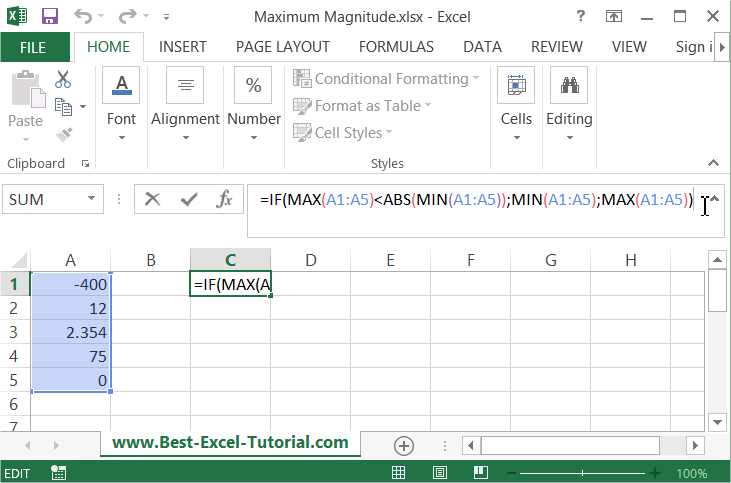#### How to Calculate Magnitude

In this Excel tutorial lesson, you will learn how to find the largest number, whether negative or positive, in a range of values. This is not obvious, but you need just one formula.

## Formula to find a magnitude

The magnitude of a number can be calculated in Excel as the absolute value of the number, which is the distance of the number from zero on the number line. Just one function is enough to determine the largest magnitude of a subset of values in Excel’s table. You should use the below formula:

=IF(MAX(A1:A100)<ABS(MIN(A1:A100));MIN(A1:A100);MAX(A1:A100))## An example of how to find the largest number

Here is the explanation of this formula:

First check IF the MAXIMUM value in the A1:A100 range is lower than ABSOLUTE of MINIMUM value. IF it is true, that means that the maximum magnitude is a MINIMUM number. Otherwise it is a MAXIMUM number. Remember that the maximum magnitude can be a negative or positive number.

Here is the whole secret of finding the maximum magnitude using the Microsoft Excel application.

## Abs formula to find a magnitude

In Excel, you can use the `ABS` function to calculate the magnitude of a number.

Here’s an example of how to calculate the magnitude of a number in Excel:

1. Open a new Excel spreadsheet.
2. In cell A1, enter the label “Number”.
3. In cell B1, enter the label “Magnitude”.
4. In cell A2, enter the number whose magnitude you want to calculate.
5. In cell B2, enter the following formula to calculate the magnitude of the number: =ABS(A2)
6. The result in cell B2 will be the magnitude of the number.
Note: You can extend this example by adding additional rows to calculate the magnitude of multiple numbers, or by using the `ABS` function in other formulas to work with the magnitude of numbers in your calculations.